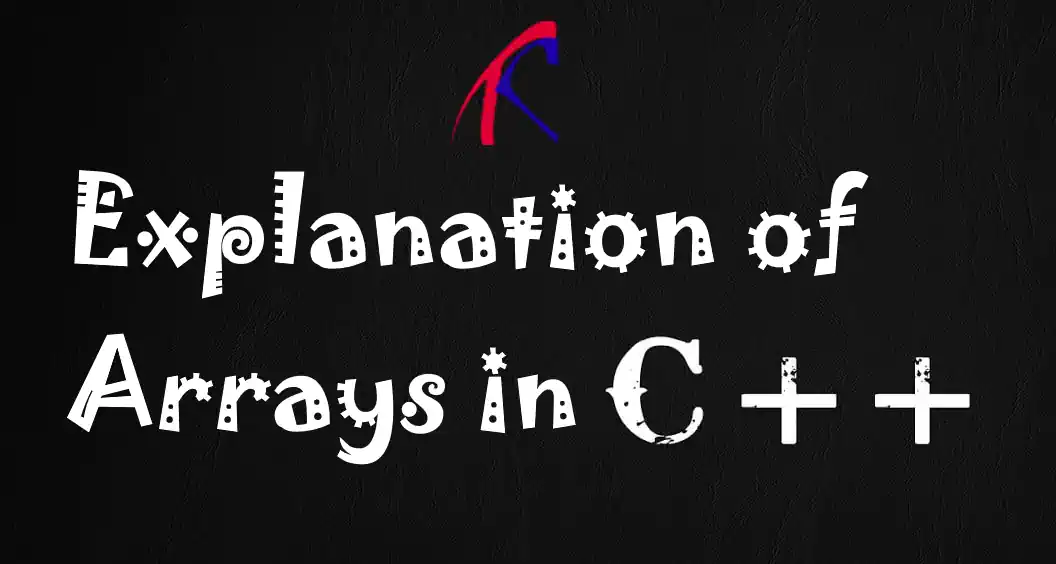## Explanation of Arrays in C++

Hello everyone, in this article we are going to talk about Arrays in C++ and some essential operations using arrays with the help of examples for better understanding.

Let's get started

Array is a collection of of same type objects. With arrays we can store same types of data in memory for processing. We can access each element by their indexes in the arrays. Arrays are very important data structure in all programming languages and for sure C++ programming language. It is very efficient to iterating the set of data and manipulating them.

#### Declaration and Initialisation of Arrays

In C++ we declare the array with starting with data type and then array name with squared brackets with the size of array. You can see an example below:
``````
double numbers;
``````
Above you can see declaration of an array with data type is double and the name of array is numbers. It will have 10 elements in it.

We can also declare and initilalise an array like below. In this way it is not required to define the size of the array within square brackets.

``````
double numbers = {10, 20, 30, 40, 50};
char myName[] = {'b', 'u', 'r', 'a', 'k'};
``````
As you can see above we have initialised the arrays with initial values. Below you can see how to access these values within array and change them by their indexes.
``````
myName = 'h';
myName = 'a';
myName = 'm';
myName = 'd';
myName = 'i';
``````
As you can see above we have declared and initialised the array with initial values, later in the code we have changed the values of array with accessing by their indexes.

#### Iterating the Arrays in C++

We can iterate the arrays with classical for loop to access to elements by the index during iterating. Below you can see an example with for loop to print the chars one by one.

``````
#include <iostream>
using namespace std;

int main() {
char myName[] = {'b', 'u', 'r', 'a', 'k'};
for (int i = 0; i < 5; i++) {
std::cout << myName[i] << endl;
}
return 0;
}
``````

Also now lets iterate the array and change the values of elements with accessing by their indexes. Below you can see an example of it.

``````
int calculateSquare(int number){
return number * number;
}

int main() {
int numbers[] = {1, 2, 3, 4, 5, 6, 7, 8, 9, 10};
for (int i = 0; i < 10; i++) {
numbers[i] = calculateSquare(numbers[i]);
}
return 0;
}
``````
In above example, the numbers in the array will be changed by their squared values.

#### Multidimensional Arrays

We can also also declare multidimensional arrays, like 2D or 3D arrays, to represent tables, matrices, or positions in space.. Here's a simple 3D array example to show the positions of a steward platform joints. It will be 6 x,y,z points to represent the position of joints.

``````
int stewartUpper = {
{4, 1, 3},
{4, 5, 6},
{7, 8, 9},
{7, 0, 12},
{9, 1, 9},
{2, 11, 9},
};
``````

We can find the maximum and minimum number of an array with iterating and checking the values in an array.

``````
#include <iostream>
using namespace std;

int main() {
int numbers = {0, 2, -120, 1234, 522, 4345, 700};
int biggest = numbers;
int smallest = numbers;
for (int i = 0; i < 7; i++) {
if (numbers[i] > biggest) {
biggest = numbers[i];
}
if (numbers[i] < smallest) {
smallest = numbers[i];
}
}
std::cout << "Biggest number is: " << biggest << endl;
std::cout << "Smallest number is: " << smallest;
return 0;
}
``````
Program output will be
``````
Biggest number is: 4345
Smallest number is: -120
``````

#### Conclusion

Arrays are very important data stricture in all programming languages and for sure for C++ also. Arrays are providing the storing the datas and accessing them to process. Understanding and using in efficient way the arrays will halp to make managings the data easier. Arrays are generally using to develop some advanced data structure for better data organising.

That is all

Burak Hamdi TUFAN

09/07/2019

14/05/2021

02/04/2022